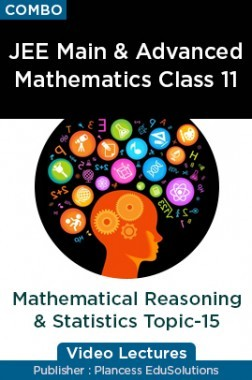•5% Off
COMBO
What is included in this combo pack?
Number Of Videos : 16

# JEE Main & Advanced Mathematics Class 11 - Mathematical Reasoning And Statistics Topic-15 Video Lectures By Plancess EduSolutions

By Plancess EduSolutions more
649 Views
Selling Price : ₹475.00
MRP : ₹500.00
You will save : ₹25.00 after 5% Discount

Save extra with 2 Offers

Get ₹ 50

Instant Cashback on the purchase of ₹ 400 or above

Product Specifications

 Publisher Plancess EduSolutions View more Author: Plancess EduSolutions Available Available in all digital devices
List of Videos
Mathematical Reasoning And Statistics - Introduction Video By Plancess 27 Mins 43 Secs
Mathematical Reasoning And Statistics - Truth Table Of Logical Connectives Video By Plancess 35 Mins 45 Secs
Mathematical Reasoning And Statistics - Illustration-I Video By Plancess 25 Mins 29 Secs
Mathematical Reasoning And Statistics - Terminologies Video By Plancess 25 Mins 37 Secs
Mathematical Reasoning And Statistics - Negation Of Connectives And Important Law Video By Plancess 34 Mins 43 Secs
Mathematical Reasoning And Statistics - Illustration And Switching Circuit Video By Plancess 28 Mins 31 Secs
Mathematical Reasoning And Statistics - Illustration-II Video By Plancess 25 Mins 28 Secs
Mathematical Reasoning And Statistics - Quantifiers And Introduction To Statistic Video By Plancess 28 Mins 7 Secs
Mathematical Reasoning And Statistics - Measure Of Central Tendency Video By Plancess 31 Mins 40 Secs
Mathematical Reasoning And Statistics - Median Video By Plancess 30 Mins 3 Secs
Mathematical Reasoning And Statistics - Quortile And Mode Video By Plancess 31 Mins 39 Secs
Mathematical Reasoning And Statistics - Symmetric Distribution And Dispersion Video By Plancess 27 Mins 19 Secs
Mathematical Reasoning And Statistics - Mean Variance Video By Plancess 35 Mins 6 Secs
Mathematical Reasoning And Statistics - Variance And Standard Deviation Video By Plancess 35 Mins 24 Secs
Mathematical Reasoning And Statistics - Illustration And Combined Standard Deviation Video By Plancess 36 Mins 53 Secs
Mathematical Reasoning And Statistics - Coefficient Of Variation Video By Plancess 21 Mins 52 Secs
View More